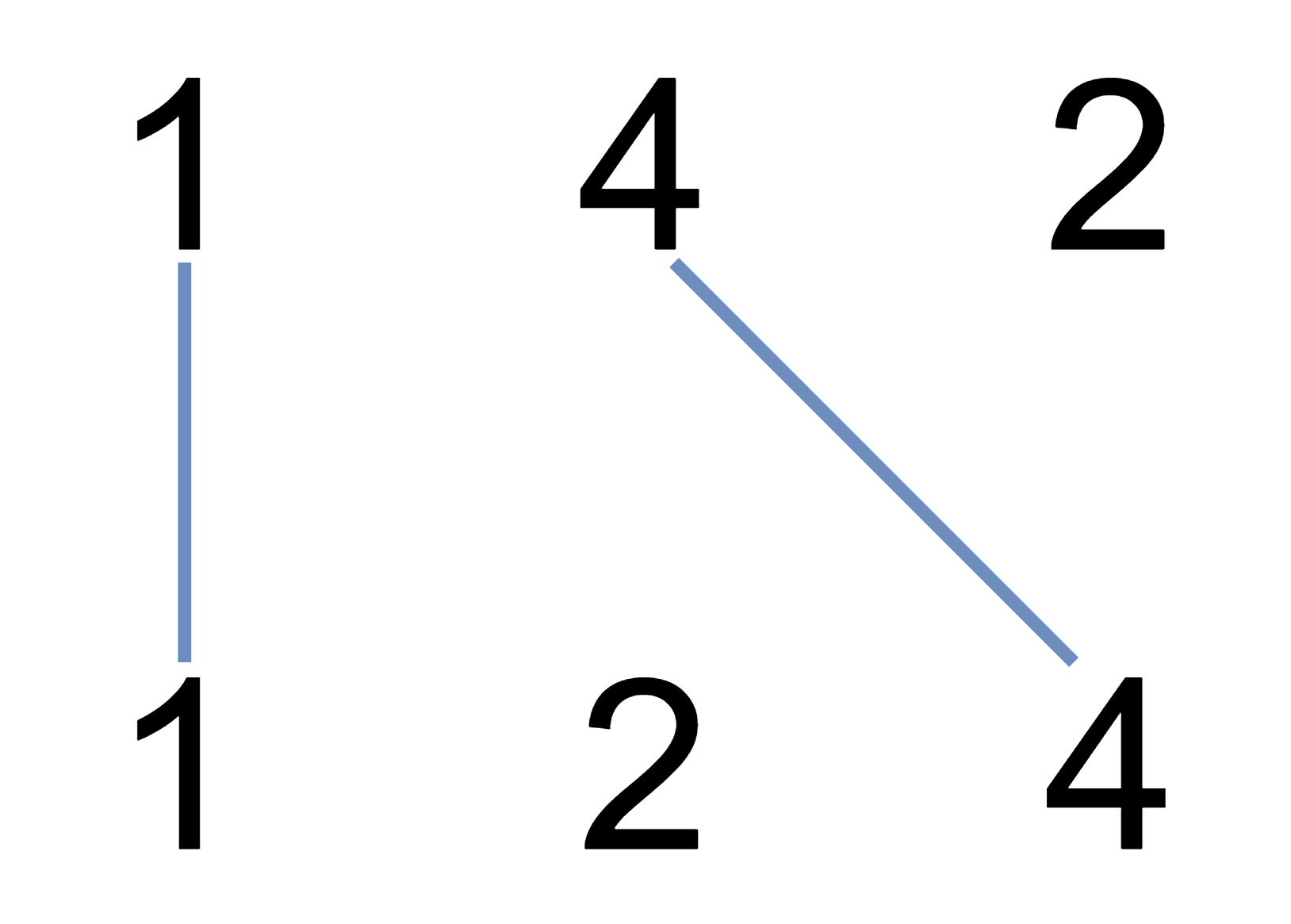# LeetCode Weekly Contest 134

https://leetcode.com/contest/weekly-contest-134

## Moving Stones Until Consecutive

Three stones are on a number line at positions a, b, and c.

Each turn, let’s say the stones are currently at positions x, y, z with x < y < z. You pick up the stone at either position x or position z, and move that stone to an integer position k, with x < k < z and k != y.

The game ends when you cannot make any more moves, ie. the stones are in consecutive positions.

When the game ends, what is the minimum and maximum number of moves that you could have made? Return the answer as an length 2 array: answer = [minimum_moves, maximum_moves]

Example 1:

Input: a = 1, b = 2, c = 5
Output: [1, 2]
Explanation: Move stone from 5 to 4 then to 3, or we can move it directly to 3.
Example 2:

Input: a = 4, b = 3, c = 2
Output: [0, 0]
Explanation: We cannot make any moves.

Note:

1. 1 <= a <= 100
2. 1 <= b <= 100
3. 1 <= c <= 100
4. a != b, b != c, c != a

## Coloring A Border

Given a 2-dimensional grid of integers, each value in the grid represents the color of the grid square at that location.

Two squares belong to the same connected component if and only if they have the same color and are next to each other in any of the 4 directions.

The border of a connected component is all the squares in the connected component that are either 4-directionally adjacent to a square not in the component, or on the boundary of the grid (the first or last row or column).

Given a square at location (r0, c0) in the grid and a color, color the border of the connected component of that square with the given color, and return the final grid.

Example 1:

Example 2:

Example 3:

Note:

1. 1 <= grid.length <= 50
2. 1 <= grid.length <= 50
3. 1 <= grid[i][j] <= 1000
4. 0 <= r0 < grid.length
5. 0 <= c0 < grid.length
6. 1 <= color <= 1000

## Uncrossed Lines

We write the integers of A and B (in the order they are given) on two separate horizontal lines.

Now, we may draw a straight line connecting two numbers A[i] and B[j] as long as A[i] == B[j], and the line we draw does not intersect any other connecting (non-horizontal) line.

Return the maximum number of connecting lines we can draw in this way.

Example 1:Example 2:

Example 3:

Note:

1. 1 <= A.length <= 500
2. 1 <= B.length <= 500
3. 1 <= A[i], B[i] <= 2000

## Escape a Large Maze

In a 1 million by 1 million grid, the coordinates of each grid square are (x, y) with 0 <= x, y < 10^6.

We start at the source square and want to reach the target square. Each move, we can walk to a 4-directionally adjacent square in the grid that isn’t in the given list of blocked squares.

Return true if and only if it is possible to reach the target square through a sequence of moves.

Example 1:

Example 2:

Note:

1. 0 <= blocked.length <= 200
2. blocked[i].length == 2
3. 0 <= blocked[i][j] < 10^6
4. source.length == target.length == 2
5. 0 <= source[i][j], target[i][j] < 10^6
6. source != target